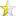Nov 14, 2014

# Split a StringIn this short article I want to share a short code about splitting a string like in PHP programming language. As we know in PHP there is a function called explode() to split a string by given delimiter (as single char or substring). For example, given a string str = "the quick brown fox" will be splitted by " " (space char). Simply we just call explode(str, " ") and the functions returns array of string {"the", "quick", "brown", "fox"}.
We can write a "PHP explode()"-like using C++, though the given delimiter is limited to a single char only. Our version of explode() returns std::vector<string> as splitted string.
Following is the definition of explode (using C++11):

 ``1234567891011121314`` ``````const vector explode(const string& s, const char& c) { string buff{""}; vector v; for(auto n:s) { if(n != c) buff+=n; else if(n == c && buff != "") { v.push_back(buff); buff = ""; } } if(buff != "") v.push_back(buff); return v; }``````

The code above just a simple function, yet well-tested with various case. Following is the example in main function:

 ``12345678`` ``````int main() { string str{"the quick brown fox jumps over the lazy dog"}; vector v{explode(str, ' ')}; for(auto n:v) cout << n << endl; return 0; }``````

will produces output:

 ``` the quick brown fox ```

This short article my be useful, hopefully.